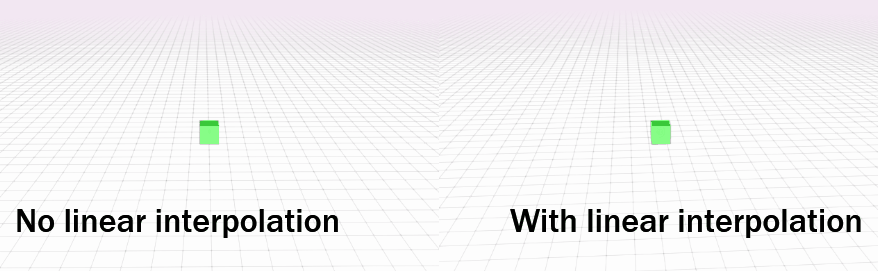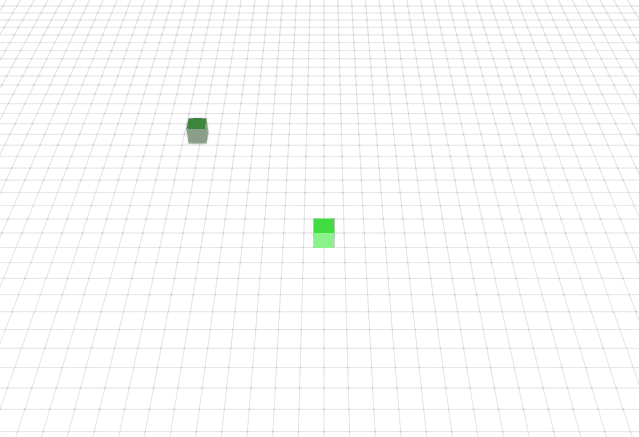# How I Implemented Client-Side Linear Interpolation

As I’m committed to developing a great game for players, I made a simple client-side linear interpolation subsystem for the new 3D multiplayer browser game I’m making.

# Background

In the context of physical movement, the transition between an object’s initial position A and a target position B can be interpolated causing a smooth-looking transition between the two positions. Linear interpolation is a means to achieve this smooth transition effect.

The following equation shows how linear interpolation determines its effect, with position being the resulting position, start being the initial position, end being the target position, and time determining the length of time of the effect.

The linear interpolation implemented as a JavaScript function which the client-side linear interpolation subsystem uses:

function lerp(start, end, time) {
return start * (1 - time) + end * time;
}


Note that the word lerp is short for linear interpolation and that the verb to lerp means to do linear interpolation.

The interpolation function can be modified to use more than a linear interpolation, but the simplicity of linear interpolation and its low cost of development has caused it to be the choice that best satisfies the program’s constraints.

Also, for documentation purposes and for creating predictive models that will be used in future game development endeavors, note that it took three hours (if I remember correctly) for me to implement this client-side linear interpolation subsystem.

# Meeting system requirements

I wanted a smooth transition between an object’s current position and its new position as given by the game server on each server transmitted game state update.

The start value will be the object’s current position value. The end value will be the server’s transmitted new position that it has determined for the game client.

The time value will be determined by the ratio between the time elapsed since the last frame and an arbitrary lerp time factor that will affect how long the movement interpolation will occur.

Using this proposed time ratio will achieve the desirable effect of having a smooth transition between object positions and thus meet system requirements.

Using these proposed utilities, theoretically, if zero time elapsed since the last frame, then the object’s position would remain the same, not changing, as evidenced by

which is desirable and meets the system requirements.

Conversely, if the time elapsed between the last frame was equal to the lerp time factor, then the object’s new position would be at the end value, as evidenced by

which is also desirable and also meets the system requirements.

## A contingency for late frames

If the time since the last frame exceeds the lerp time factor, then the position is simply instantaneously changed instead of being linearly interpolated. A simple if conditional is used to determine this.

if (time_since_last_frame > lerp_time_factor) // do not use linear interpolation
position = server_updated_position;


# Implementation

Below is a stylized implementation of what the simple client-side linear interpolation subsystem uses.

if (time_since_last_frame <= lerp_time_factor) { // use linear interpolation
position = lerp(position, server_updated_position, time_since_last_frame / lerp_time_factor);
} else { // do not use linear interpolation
position = server_updated_position;
}


Note that the physical movement in the actual game is actually frame rate independent and has been presented here as being dependent on the frame rate for the reader’s sake.

# The results

The picture shown below is a comparison between no linear interpolation being used and with linear interpolation being used. The left side shows jerky or disconnected movement as no linear interpolation is being used there while the right side shows a smooth transition between positions, per system requirements, with linear interpolation being used.# Client-side prediction and rubber banding

So as to not wait on the game server for new coordinates to be transmitted as network latency can cause delays in client-server communication, as the player issues a movement command, the game client immediately changes the player’s position before it receives the correct position from the game server, predicting what it will be for the player’s benefit. The linear interpolation subsystem will then smoothly correct the player’s position during the next frame when the client receives the actual position from the server.

In conjunction with the use of this client-side prediction, when the game client loses its connection to the game server, a rubber banding effect occurs during player movement commands where the player’s avatar behaves as if it was rubber banded to its position due to not receiving server position updates and thus always lerping between its current position and its predicted position until the connection to the server is reestablished.Note that this rubber banding effect occurs and is less exaggerated during periods of network subsystem jitter. This is to be expected as it is common for networked multiplayer games that use linear interpolation.

January 20, 2019